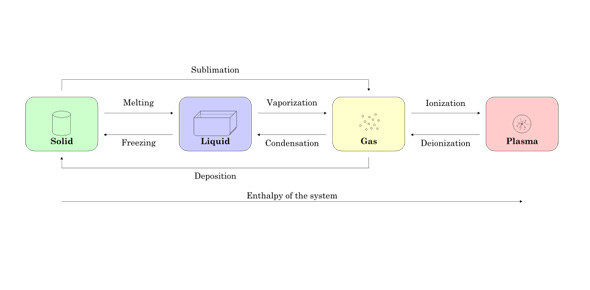# Quiz On States Of Matter & Phase Changes

10 Questions | Attempts: 176SettingsThe following will test your understanding of the states of matter and phase changes.

• 1.
Which of the following could solid ice become if heat energy is added? (Check all that apply)
• A.

Solid Ice

• B.

Liquid Water

• C.

Vapor

• D.

None of the above, energy needs to be removed

• 2.
If isopropyl alcohol is a liquid at room temperature (25 degrees Celsius), which of the following must its boiling point be?
• A.

0 Degrees Celsius

• B.

20 Degrees Celsius

• C.

25 Degrees Celsius

• D.

82 Degrees Celsius

• 3.
Which of the following terms identifies the change from a liquid to solid?
• A.

Melting

• B.

Solidification

• C.

Boiling

• D.

Sublimation

• 4.
Oxygen becomes a gas at -183 degrees Celsius. We would call this temperature?
• A.

Boiling point

• B.

Evaporating point

• C.

Sublimation point

• D.

Freezing point

• 5.
The following occurs when heat energy is removed:Gas ---(A)-->    Liquid  ---(B)--->    SolidWhat would be the phase changes for A and B respectfully?
• A.

Melting, Evaporating

• B.

Melting, Condensation

• C.

Condensation, Freezing

• D.

Condensation, Melting

• E.

Boiling, Freezing

• 6.
Examine the following sequence of phase changes:Solid --->  Liquid --->  GasWould we need to ADD or REMOVE heat energy?
• A.

• B.

Remove heat energy

• C.

Neither

• 7.
Freezing is to solid, as sublimation is to ______________________
• A.

Solid

• B.

Liquid

• C.

Gas

• 8.
If chemical "X" had the following physical properties:Melting Point =   - 32 Degrees CelsiusBoiling Point =    -11  Degrees Celsiuswhat state of matter would you find Chemical "X" if the temperature was 0 Degrees Celsius?
• A.

Solid

• B.

Liquid

• C.

Gas

• 9.
When isopropyl alcohol was spilled over the counter top and quickly disappeared, what phase change occurred?
• A.

Evaporation

• B.

Boiling

• C.

Condensation

• D.

Sublimation

• 10.
A student noticed frost was on the ground at 6:30 a.m. but noticed it disappeared by 8:30 a.m. What phase change was responsible for turning the frost into water vapor?
• A.

Melting

• B.

Evaporation

• C.

Sublimation

• D.

Deposition

## Related TopicsBack to top
×

Wait!
Here's an interesting quiz for you.